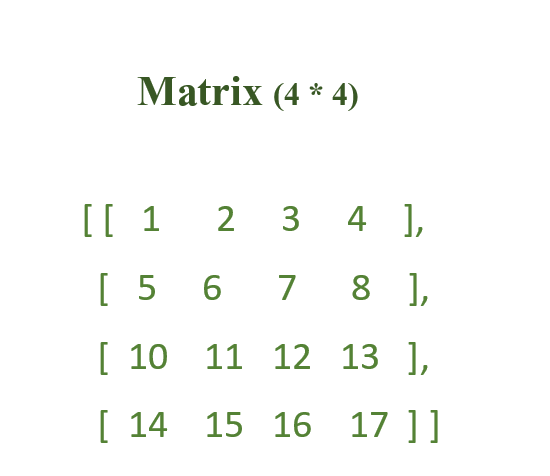GFG App
Open AppBrowser
Continue

A matrix is a two-dimensional array that consists of rows and columns. It is an arrangement of elements in horizontal or vertical lines of entries.Example of a 4×4 matrix

### Characteristics of Matrix:

• Dimensions: The dimensions of a matrix are given by the number of rows and columns. A matrix with m rows and n columns is said to have dimensions m x n.
• Elements: The elements of a matrix are the values stored in each cell of the matrix.
• Transpose: The transpose of a matrix is obtained by interchanging its rows and columns.
• Determinant: The determinant of a square matrix is a scalar value that can be calculated using its elements.
• Inverse: The inverse of a square matrix is a matrix that, when multiplied by the original matrix, results in the identity matrix.

### Different types of Matrix :

Here are some common types of matrices:

• Square matrix: A square matrix is a matrix in which the number of rows and columns are equal. In other words, it has the same number of rows as columns. For example, a 3×3 matrix is a square matrix.
• Identity matrix: An identity matrix is a square matrix in which all the diagonal elements are 1 and all other elements are 0.
• Diagonal matrix: A diagonal matrix is a square matrix in which all the non-diagonal elements are 0.
• Upper triangular matrix: An upper triangular matrix is a square matrix in which all the elements below the main diagonal (the diagonal from the top left corner to the bottom right corner) are 0.
• Lower triangular matrix: A lower triangular matrix is a square matrix in which all the elements above the main diagonal are 0.
• Sparse matrix: A sparse matrix is a matrix in which the majority of the elements are 0. Sparse matrices are often represented using compressed sparse row (CSR) or compressed sparse column (CSC) formats to save space and optimize performance in algorithms that work with such matrices.

### Applications of Matrix:

• Graph theory: Matrices can be used to represent graphs and networks. For example, the adjacency matrix of a graph is a matrix that describes the connections between nodes in the graph.
• Image processing: Matrices are commonly used in image processing to represent images as matrices of pixel values.
• Optimization: Matrices are used in optimization problems, such as linear programming and quadratic programming, to represent the objective function and constraints of the problem.
• Machine learning:  Matrices are used to represent training data and model parameters, and matrix operations are used to perform model training and prediction.

• Compact representation: Matrices can represent large amounts of data in a compact form, making it easier to store and manipulate data efficiently.
• Easy to manipulate: Matrices can be manipulated easily using various operations, such as addition, subtraction, multiplication, and transpose.
• Versatile: Matrices can be used to represent a wide range of data types, such as images, graphs, and networks.
• Parallel computing: Matrix operations can be easily parallelized, which means that they can be computed simultaneously on multiple processors.